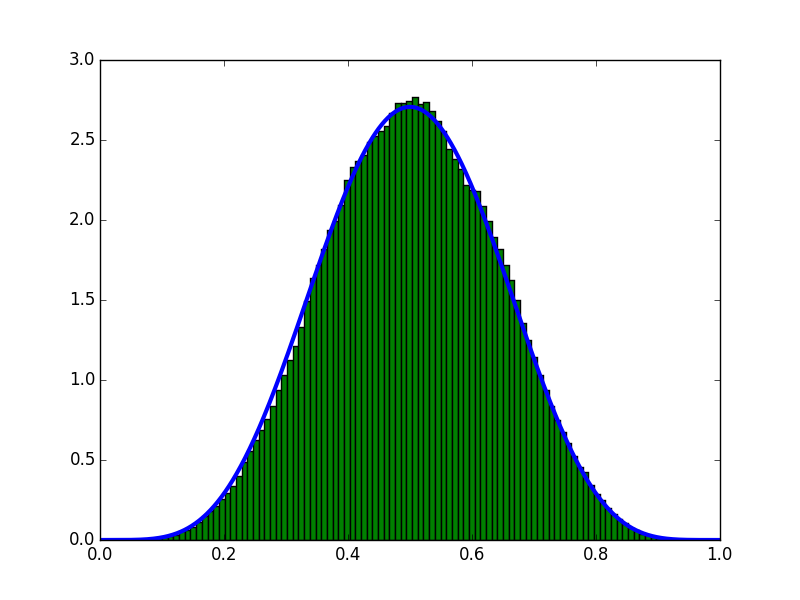# Estimate the probability that a coin will appear on the table using MCMC

## Overview

For studying MCMC. As the simplest example, the posterior distribution of parameters in the Bernoulli process was calculated using MCMC and beta distribution.

## MCMC overview

MCMC (Markov Chain Monte Carlo) Method in Bayesian Statistics Is a method of finding the posterior distribution using random numbers.

The posterior distribution uses Bayes' theorem

Posterior distribution = (likelihood * prior distribution) / (probability of obtaining data)

Can be obtained by. The problem here is that the probability that the denominator data on the right side can be obtained The probability that the data can be obtained p (D) can be obtained by integrating and eliminating using the parameter $\ theta$. However, as the number of dimensions of the parameter increases, multiple multiple integrals must be performed. It is possible to obtain an approximate integral value using the static Monte Carlo method, but the accuracy decreases as the number of dimensions increases. Therefore, MCMC gave up calculating the probability of obtaining data and estimated the posterior distribution only from the likelihood and prior distribution.

MCMC allows you to get a sample proportional to the value of the function. Therefore, if the likelihood * prior distribution is sampled by MCMC and normalized so that the integral value becomes 1, it becomes the posterior distribution.

This time, we will find the probability density function that the coin appears from the data obtained by throwing the coin several times, which is the easiest case for studying.

## Likelihood

Let the probability of coins appearing as $\ mu$ Suppose a coin toss obtains a {0,1} set D in an independent trial.

D={0,1,...,0,1}

At this time the likelihood is

p(D|\mu)=\prod_{n} \mu^{x_{n}} (1-\mu)^{1-x_{n}}

## Prior distribution

Prior distributions take uniform values as they do not have any prior information about coins Let p ($\ mu$) = 1.

## Ex-post distribution

### Use MCMC

Sampling is performed by looping a predetermined number of times according to the following procedure.

1. Select the initial value of the parameter $\ mu$
2. Randomly move $\ mu$ a small amount to get $\ mu_ {new}$
3. If (likelihood * prior distribution) becomes large as a result of moving, update $\ mu$ to $\ mu_ {new}$ for the moved value → go to 2.
4. (Likelihood*Prior distribution)If becomes smaller(P(D|\mu_{new})P( \mu_{new})/(P(D|\mu)P( \mu)With the probability of\muUpdate → 2.What

The histogram of the obtained sample is integrated and normalized to 1 to obtain the posterior distribution.

### Find analytically

Since this model is a Bernoulli process, assuming a beta distribution as a prior distribution, The posterior distribution can be obtained simply by updating the parameters of the beta distribution.

Let m be the number of times the coin appears on the front, l be the number of times the coin appears on the back, and let a and b be predetermined parameters.

$p(\mu|m,l,a,b) = beta(m+a,l+b)$

Will be. If the prior distribution is an informationless prior distribution, set a = b = 1.

## program

I wrote it in python. I think it will work if you have anaconda. It's not fast at all because it emphasizes readability.

# coding:utf-8
from scipy.stats import beta
import matplotlib.pyplot as plt
import numpy as np

def priorDistribution(mu):
if 0 < mu < 1:
return 1
else:
return 10 ** -9

def logLikelihood(mu, D):
if 0 < mu < 1:
return np.sum(D * np.log(mu) + (1 - D) * np.log(1 - mu))
else:
return -np.inf

np.random.seed(0)
sample = 0.5
sample_list = []
burnin_ratio = 0.1
N = 10
# make data
D = np.array([1, 0, 1, 1, 0, 1, 0, 1, 0, 0])
l = logLikelihood(sample, D) + np.log(priorDistribution(sample))
# Start MCMC
for i in range(10 ** 6):
# make candidate sample
sample_candidate = np.random.normal(sample, 10 ** -2)
l_new = logLikelihood(sample_candidate, D) + \
np.log(priorDistribution(sample_candidate))
# decide accept
if min(1, np.exp(l_new - l)) > np.random.random():
sample = sample_candidate
l = l_new
sample_list.append(sample)
sample_list = np.array(sample_list)
sample_list = sample_list[int(burnin_ratio * N):]

# calc beta
x = np.linspace(0, 1, 1000)
y = beta.pdf(x, sum(D) + 1, N - sum(D) + 1)

plt.plot(x, y, linewidth=3)
plt.hist(sample_list, normed=True, bins=100)
plt.show()



## Execution result

Estimated on the condition that 10 samples (5 on the front and 5 on the back) were obtained. The blue line is the graph obtained analytically, and the histogram is the graph obtained by MCMC. If you look at the graph, you can see that it is estimated well.## Summary

This time, the parameters of the Bernoulli distribution were obtained by handwriting with python and MCMC, which is a very inefficient method. It was a problem setting that could not receive the benefit of MCMC with no information prior distribution and one variable with domain [0,1], but if the likelihood (model) can be defined, the posterior distribution can be thrown to a personal computer. I was able to imagine that I could get it.

When actually using MCMC, it is a good idea to use an optimized library such as Stan or PyMC.

By playing with different prior distributions and parameters, you may be able to understand the unique properties of Bayesian estimation, such as the larger the number of observed data, the smaller the width of the distribution and the less the influence of the prior distribution.

## References

An introduction to statistical modeling for data analysis http://hosho.ees.hokudai.ac.jp/~kubo/stat/2013/ou3/kubostat2013ou3.pdf http://tjo.hatenablog.com/entry/2014/02/08/173324